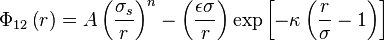# Soft sphere attractive Yukawa model

(diff) ← Older revision | Latest revision (diff) | Newer revision → (diff)$\Phi_{12}\left( r \right) = A\left( \frac{\sigma_s}{r} \right)^n - \left( \frac{ \epsilon \sigma }{r}\right) \exp \left[- \kappa \left( \frac{r}{\sigma} - 1 \right) \right]$